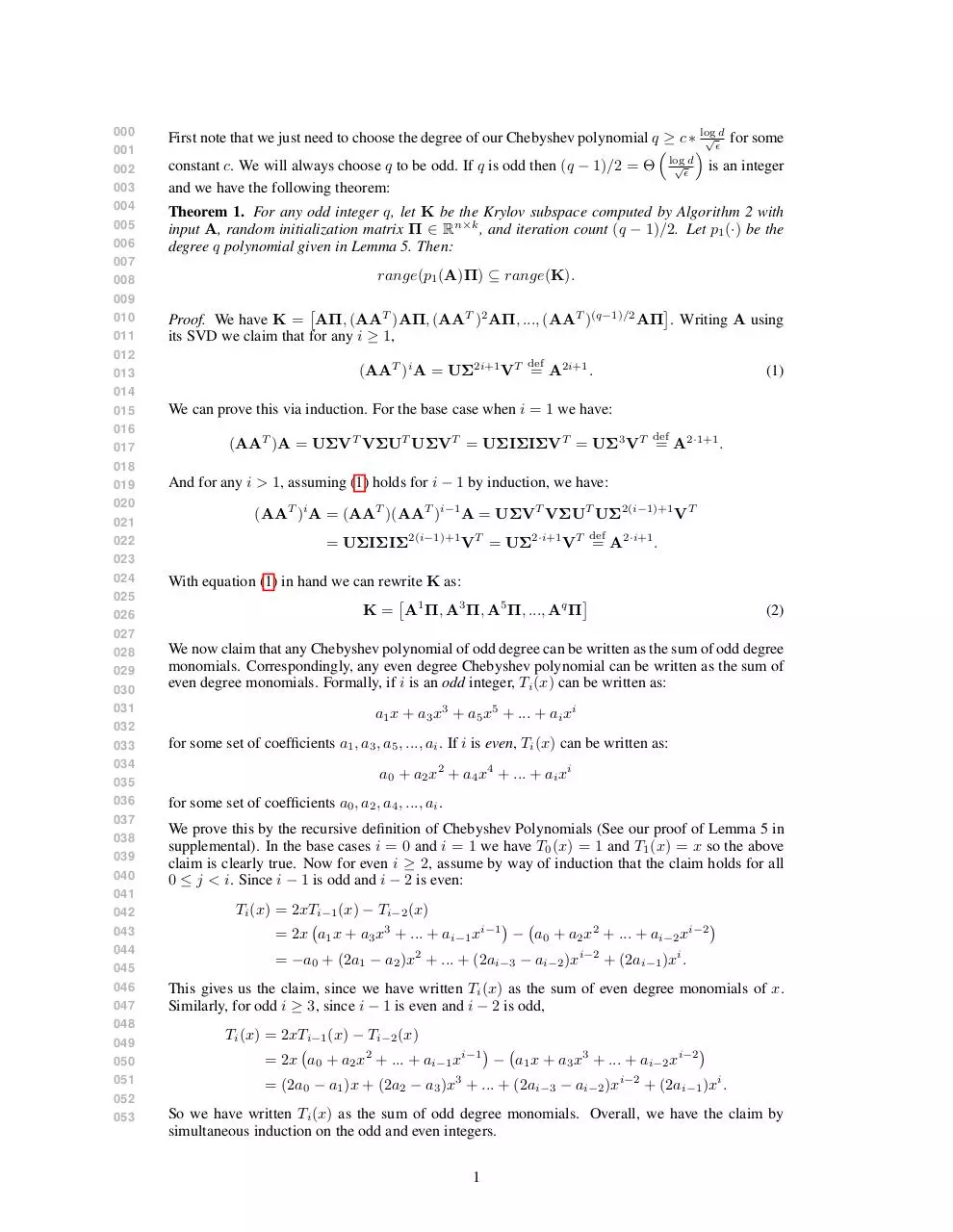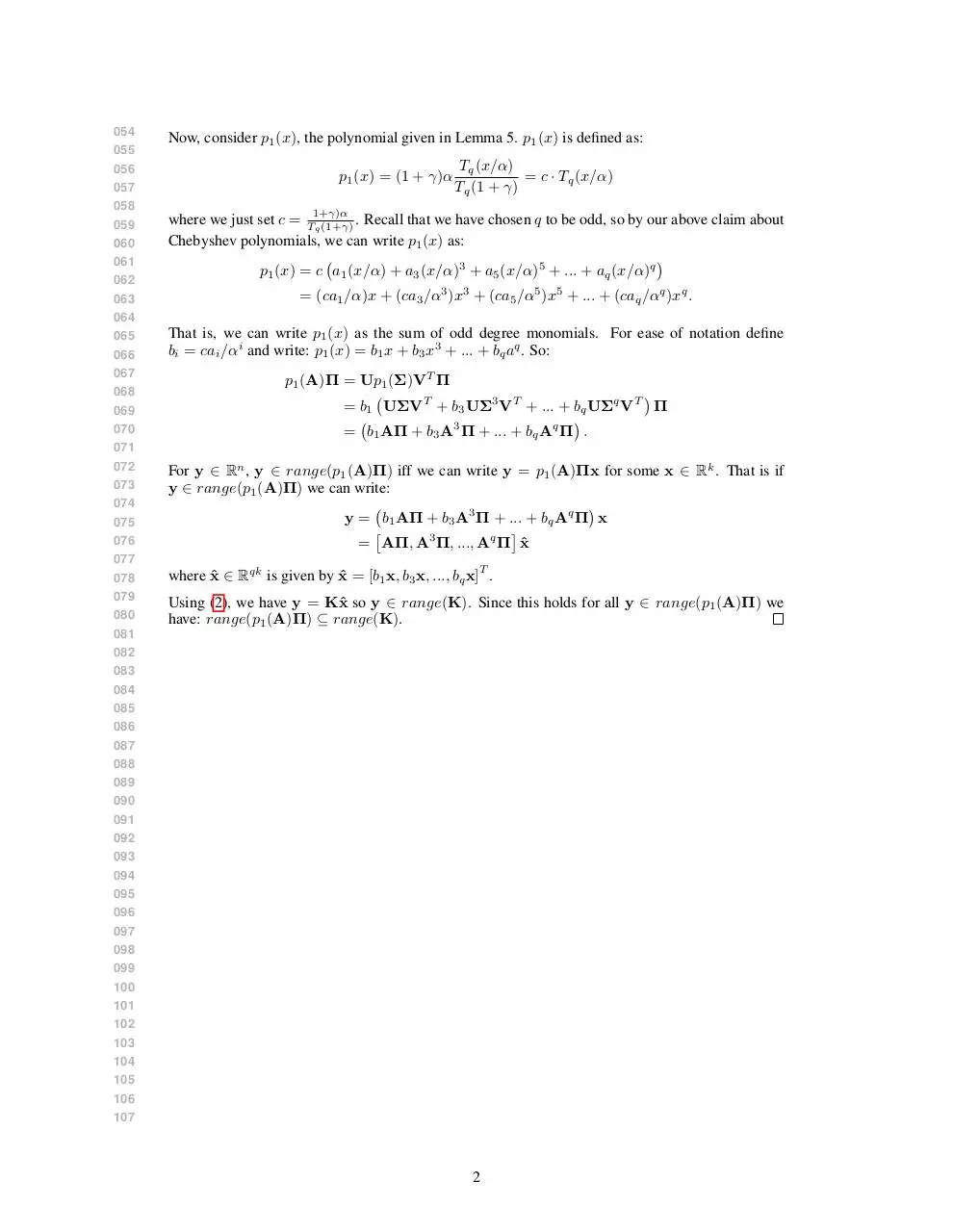# oddMonomialProof .pdf

### File information

This PDF 1.5 document has been generated by LaTeX with hyperref package / pdfTeX-1.40.14, and has been sent on pdf-archive.com on 07/08/2015 at 00:05, from IP address 128.30.x.x. The current document download page has been viewed 492 times.
File size: 131.88 KB (2 pages).
Privacy: public file

### Document preview

000
001
002
003
004
005
006
007
008
009
010
011
012
013
014
015
016
017
018
019
020
021
022
023
024
025
026
027
028
029
030
031
032
033
034
035
036
037
038
039
040
041
042
043
044
045
046
047
048
049
050
051
052
053

√ d for some
First note that we just need to choose the degree of our Chebyshev polynomial q ≥ c ∗ log
 

√ d is an integer
constant c. We will always choose q to be odd. If q is odd then (q − 1)/2 = Θ log

and we have the following theorem:

Theorem 1. For any odd integer q, let K be the Krylov subspace computed by Algorithm 2 with
input A, random initialization matrix Π ∈ Rn×k , and iteration count (q − 1)/2. Let p1 (·) be the
degree q polynomial given in Lemma 5. Then:
range(p1 (A)Π) ⊆ range(K).


Proof. We have K = AΠ, (AAT )AΠ, (AAT )2 AΠ, ..., (AAT )(q−1)/2 AΠ . Writing A using
its SVD we claim that for any i ≥ 1,
def

(AAT )i A = UΣ2i+1 VT = A2i+1 .

(1)

We can prove this via induction. For the base case when i = 1 we have:
def

(AAT )A = UΣVT VΣUT UΣVT = UΣIΣIΣVT = UΣ3 VT = A2·1+1 .
And for any i &gt; 1, assuming (1) holds for i − 1 by induction, we have:
(AAT )i A = (AAT )(AAT )i−1 A = UΣVT VΣUT UΣ2(i−1)+1 VT
def

= UΣIΣIΣ2(i−1)+1 VT = UΣ2·i+1 VT = A2·i+1 .
With equation (1) in hand we can rewrite K as:


K = A1 Π, A3 Π, A5 Π, ..., Aq Π

(2)

We now claim that any Chebyshev polynomial of odd degree can be written as the sum of odd degree
monomials. Correspondingly, any even degree Chebyshev polynomial can be written as the sum of
even degree monomials. Formally, if i is an odd integer, Ti (x) can be written as:
a1 x + a3 x3 + a5 x5 + ... + ai xi
for some set of coefficients a1 , a3 , a5 , ..., ai . If i is even, Ti (x) can be written as:
a0 + a2 x2 + a4 x4 + ... + ai xi
for some set of coefficients a0 , a2 , a4 , ..., ai .
We prove this by the recursive definition of Chebyshev Polynomials (See our proof of Lemma 5 in
supplemental). In the base cases i = 0 and i = 1 we have T0 (x) = 1 and T1 (x) = x so the above
claim is clearly true. Now for even i ≥ 2, assume by way of induction that the claim holds for all
0 ≤ j &lt; i. Since i − 1 is odd and i − 2 is even:
Ti (x) = 2xTi−1 (x) − Ti−2 (x)


= 2x a1 x + a3 x3 + ... + ai−1 xi−1 − a0 + a2 x2 + ... + ai−2 xi−2
= −a0 + (2a1 − a2 )x2 + ... + (2ai−3 − ai−2 )xi−2 + (2ai−1 )xi .
This gives us the claim, since we have written Ti (x) as the sum of even degree monomials of x.
Similarly, for odd i ≥ 3, since i − 1 is even and i − 2 is odd,
Ti (x) = 2xTi−1 (x) − Ti−2 (x)


= 2x a0 + a2 x2 + ... + ai−1 xi−1 − a1 x + a3 x3 + ... + ai−2 xi−2
= (2a0 − a1 )x + (2a2 − a3 )x3 + ... + (2ai−3 − ai−2 )xi−2 + (2ai−1 )xi .
So we have written Ti (x) as the sum of odd degree monomials. Overall, we have the claim by
simultaneous induction on the odd and even integers.
1

054
055
056
057
058
059
060
061
062
063
064
065
066
067
068
069
070
071
072
073
074
075
076
077
078
079
080
081
082
083
084

Now, consider p1 (x), the polynomial given in Lemma 5. p1 (x) is defined as:
p1 (x) = (1 + γ)α

Tq (x/α)
= c · Tq (x/α)
Tq (1 + γ)

where we just set c = T1+γ)α
. Recall that we have chosen q to be odd, so by our above claim about
q (1+γ)
Chebyshev polynomials, we can write p1 (x) as:

p1 (x) = c a1 (x/α) + a3 (x/α)3 + a5 (x/α)5 + ... + aq (x/α)q
= (ca1 /α)x + (ca3 /α3 )x3 + (ca5 /α5 )x5 + ... + (caq /αq )xq .
That is, we can write p1 (x) as the sum of odd degree monomials. For ease of notation define
bi = cai /αi and write: p1 (x) = b1 x + b3 x3 + ... + bq aq . So:
p1 (A)Π = Up1 (Σ)VT Π

= b1 UΣVT + b3 UΣ3 VT + ... + bq UΣq VT Π

= b1 AΠ + b3 A3 Π + ... + bq Aq Π .
For y ∈ Rn , y ∈ range(p1 (A)Π) iff we can write y = p1 (A)Πx for some x ∈ Rk . That is if
y ∈ range(p1 (A)Π) we can write:

y = b1 AΠ + b3 A3 Π + ... + bq Aq Π x


= AΠ, A3 Π, ..., Aq Π x
ˆ
T

where x
ˆ ∈ Rqk is given by x
ˆ = [b1 x, b3 x, ..., bq x] .
Using (2), we have y = Kˆ
x so y ∈ range(K). Since this holds for all y ∈ range(p1 (A)Π) we
have: range(p1 (A)Π) ⊆ range(K).

085
086
087
088
089
090
091
092
093
094
095
096
097
098
099
100
101
102
103
104
105
106
107

2oddMonomialProof.pdf (PDF, 131.88 KB)

### Share on social networks

#### HTML Code

Copy the following HTML code to share your document on a Website or Blog